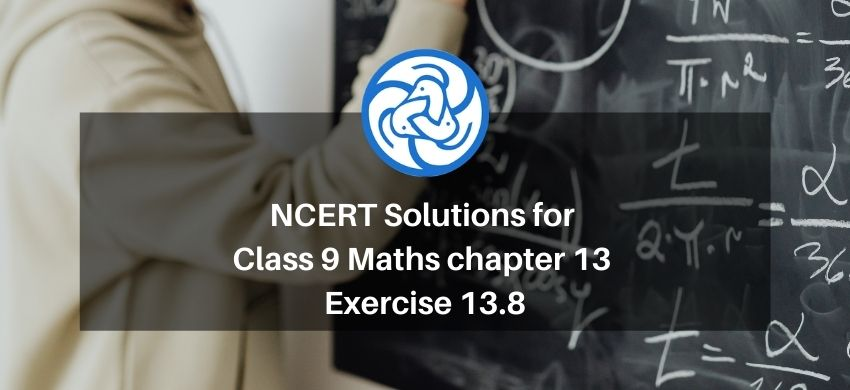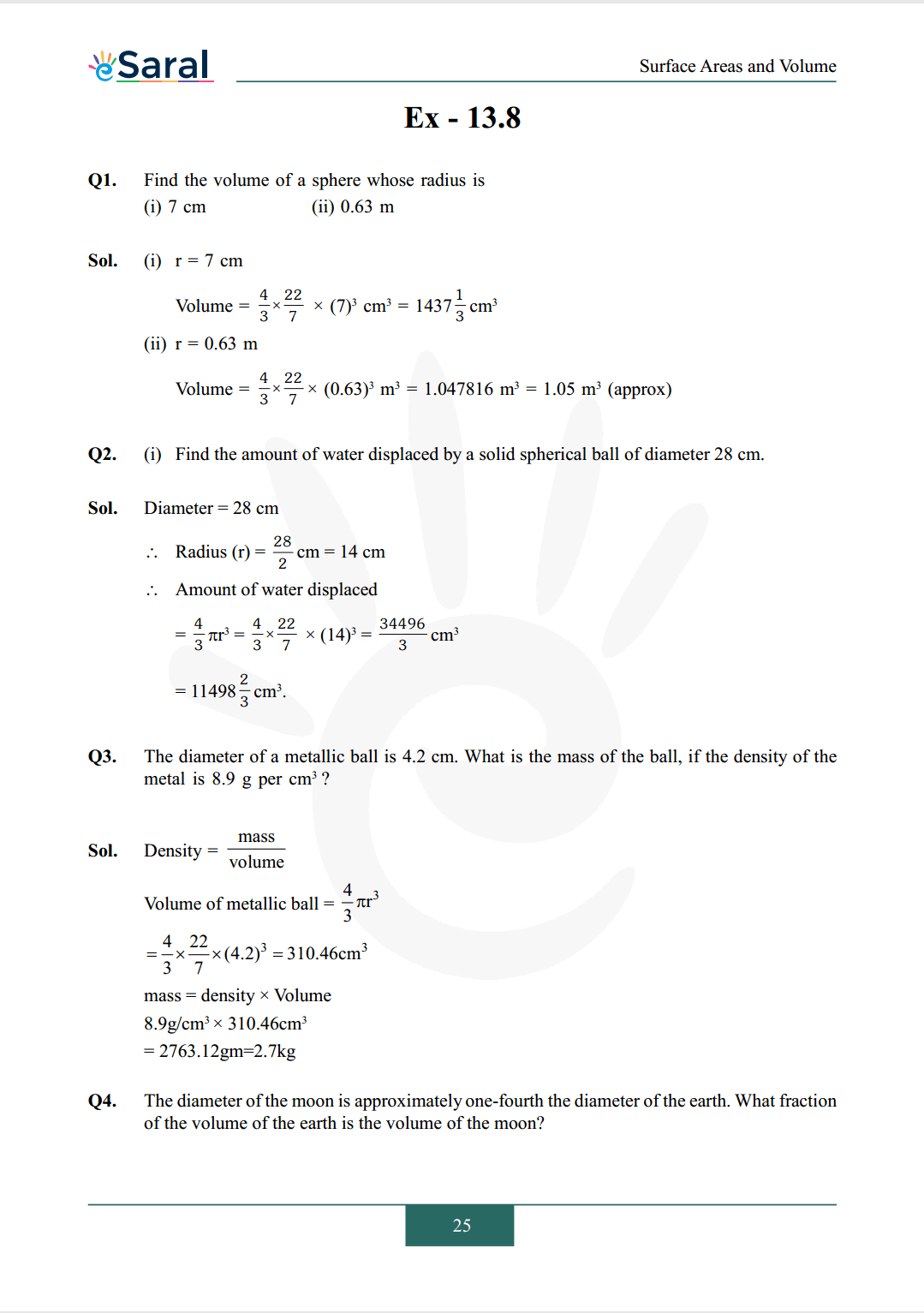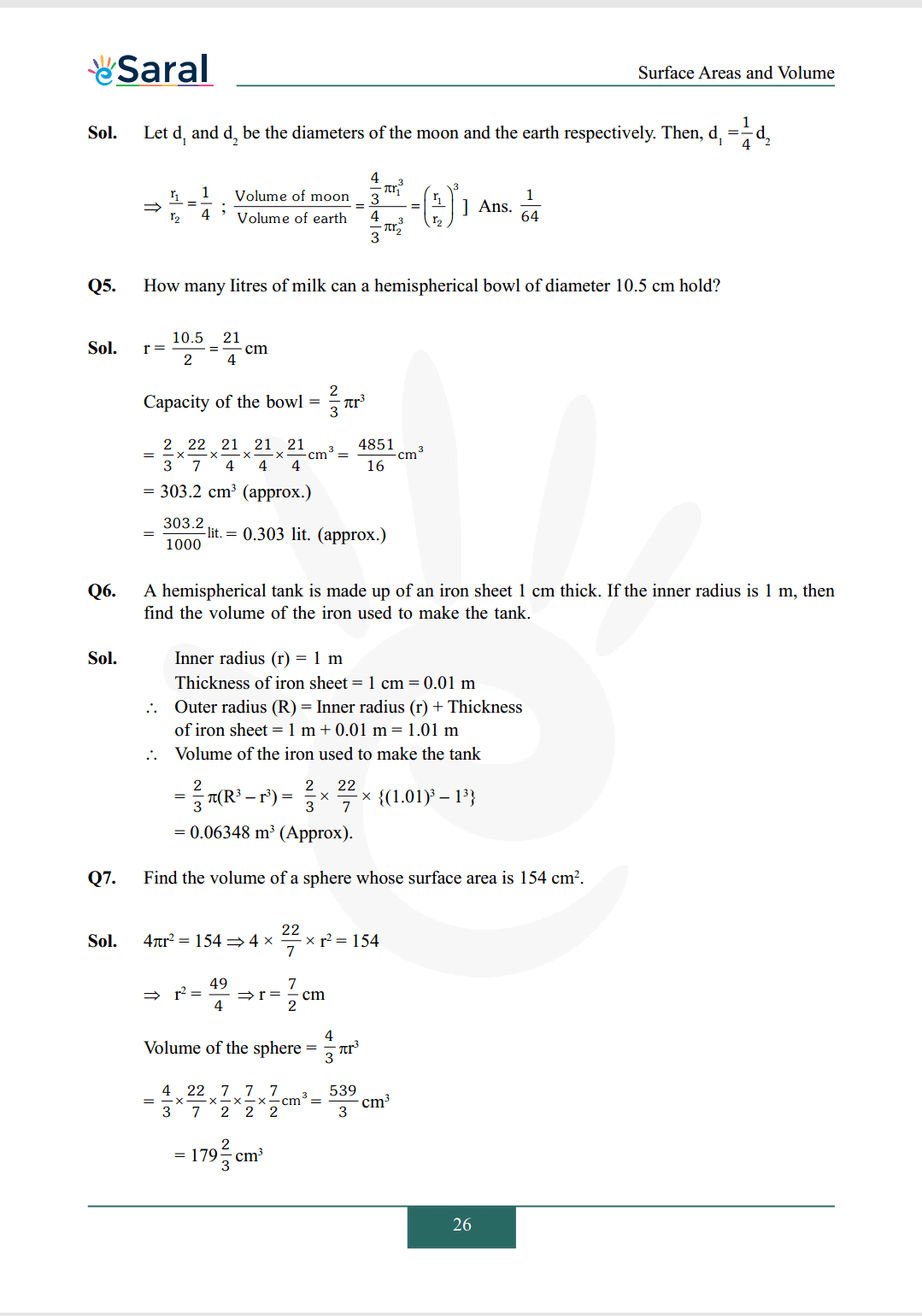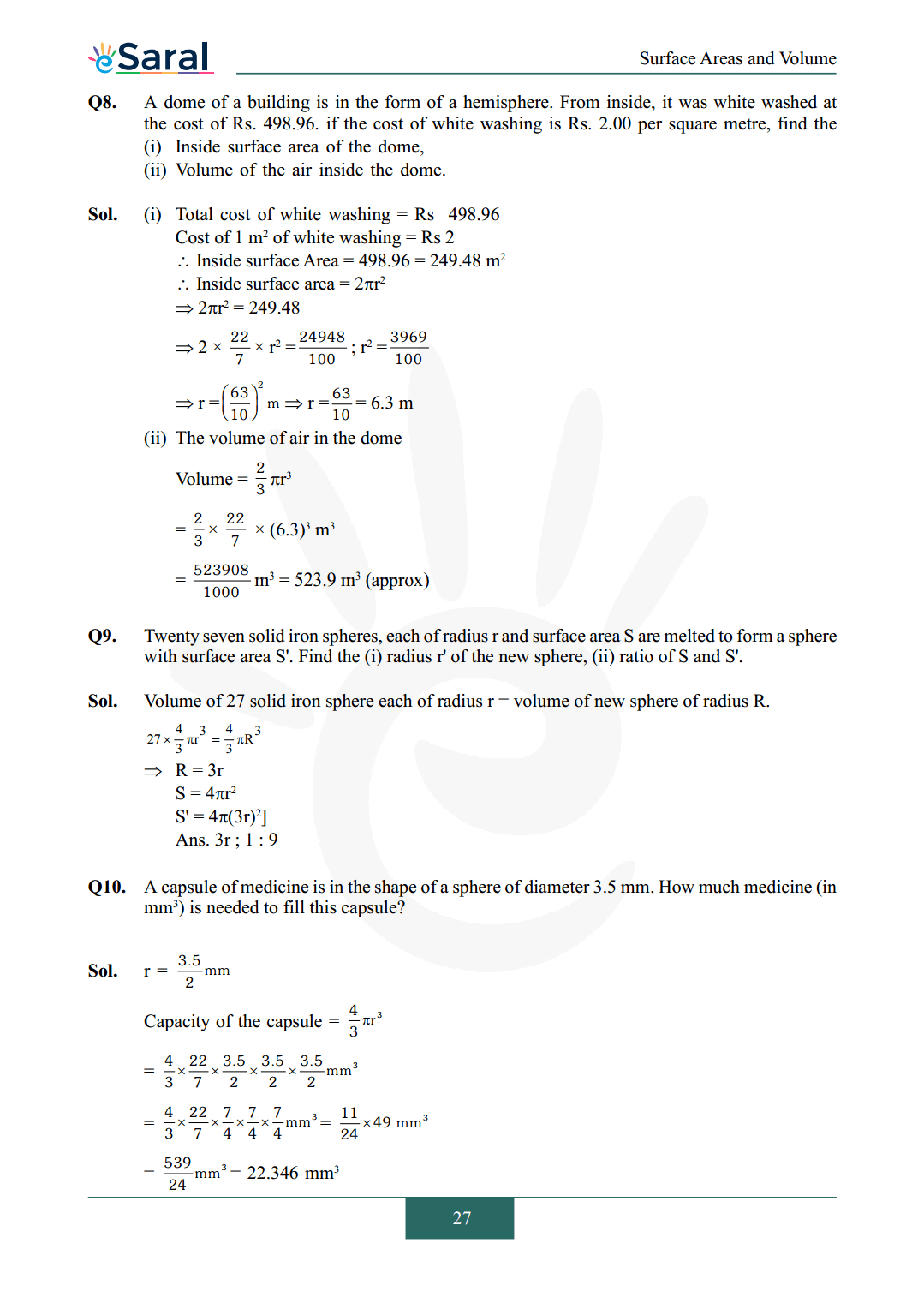Most Affordable JEE | NEET | 8,9,10 Preparation by Kota's Top IITian Doctor Faculties

# NCERT Solutions for Class 9 Maths chapter 13 Exercise 13.8 - Surface Areas and VolumesHey, are you a class 9 Student and Looking for Ways to Download NCERT Solutions for Class 9 Maths chapter 13 Exercise 13.8? If Yes then you are at the right place.

Here we have listed Class 9 maths chapter 13 exercise 13.8 solutions in PDF that is prepared by Kota’s top IITian’s Faculties by keeping Simplicity in mind.

If you want to score high in your class 9 Maths Exam then it is very important for you to have a good knowledge of all the important topics, so to learn and practice those topics you can use eSaral NCERT Solutions.

So, without wasting more time Let’s start.

### Download The PDF of NCERT Solutions for Class 9 Maths chapter 13 Exercise 13.8 "Surface Areas and Volumes"#### All Questions of Chapter 13 Exercise 13.8

Once you complete the chapter 13 then you can revise Ex. 13.8 by solving following questions

Q1. Find the volume of a sphere whose radius is
(i) $7 \mathrm{~cm}$
(ii) $0.63 \mathrm{~m}$

Q2. (i) Find the amount of water displaced by a solid spherical ball of diameter 28 cm.

Q3. The diameter of a metallic ball is $4.2 \mathrm{~cm}$. What is the mass of the ball, if the density of the metal is $8.9 \mathrm{~g}$ per $\mathrm{cm}^{3}$ ?

Q4. The diameter of the moon is approximately one-fourth the diameter of the earth. What fraction of the volume of the earth is the volume of the moon?

Q5. How many Iitres of milk can a hemispherical bowl of diameter 10.5 cm hold?

Q6. A hemispherical tank is made up of an iron sheet 1 cm thick. If the inner radius is 1 m, then find the volume of the iron used to make the tank.

Q7. Find the volume of a sphere whose surface area is $154 \mathrm{~cm} ^ {2}$.

Q8. A dome of a building is in the form of a hemisphere. From inside, it was white washed at the cost of Rs. 498.96. if the cost of white washing is Rs. $2.00$ per square metre, find the
(i) Inside surface area of the dome,
(ii) Volume of the air inside the dome.

Q9. Twenty seven solid iron spheres, each of radius $\mathrm{r}$ and surface area $\mathrm{S}$ are melted to form a sphere with surface area $\mathrm{S}^{\prime}$. Find the
(i) radius $\mathrm{r}^{\prime}$ of the new sphere,
(ii) ratio of $\mathrm{S}$ and $\mathrm{S}$ '.

Q10. A capsule of medicine is in the shape of a sphere of diameter $3.5 \mathrm{~mm}$. How much medicine (in $\mathrm{mm}^{3}$ ) is needed to fill this capsule?

NCERT Class 9 Maths Book PDF

NCERT Class 9 Maths Exemplar PDF

Complete Solutions for Class 9 Maths chapter 13 PDF